# Precalculus : Inverse Trigonometric Functions

## Example Questions

2 Next →

### Example Question #11 : Inverse Trigonometric Functions

Approximate the following: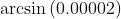is closest in value to which of the following?

Possible Answers:

0.2

4

1

2

0

Correct answer:

0

Explanation:

This problem is quite manageable with knowledge of the unit circle: the value is extremely close to zero, of which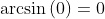always, so the only reasonable estimation of this value is 0.

### Example Question #12 : Inverse Trigonometric Functions

Evaluate: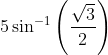Possible Answers: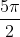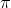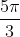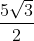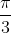Correct answer:Explanation: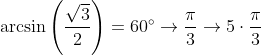and so the credited answer is.

2 Next →

### All Precalculus Resources